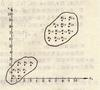kmeans 订阅
k均值聚类算法（k-means clustering algorithm）是一种迭代求解的聚类分析算法，其步骤是，预将数据分为K组，则随机选取K个对象作为初始的聚类中心，然后计算每个对象与各个种子聚类中心之间的距离，把每个对象分配给距离它最近的聚类中心。聚类中心以及分配给它们的对象就代表一个聚类。每分配一个样本，聚类的聚类中心会根据聚类中现有的对象被重新计算。这个过程将不断重复直到满足某个终止条件。终止条件可以是没有（或最小数目）对象被重新分配给不同的聚类，没有（或最小数目）聚类中心再发生变化，误差平方和局部最小。 展开全文k均值聚类算法（k-means clustering algorithm）是一种迭代求解的聚类分析算法，其步骤是，预将数据分为K组，则随机选取K个对象作为初始的聚类中心，然后计算每个对象与各个种子聚类中心之间的距离，把每个对象分配给距离它最近的聚类中心。聚类中心以及分配给它们的对象就代表一个聚类。每分配一个样本，聚类的聚类中心会根据聚类中现有的对象被重新计算。这个过程将不断重复直到满足某个终止条件。终止条件可以是没有（或最小数目）对象被重新分配给不同的聚类，没有（或最小数目）聚类中心再发生变化，误差平方和局部最小。

1967年

James MacQueen

K均值聚类算法

k-means clustering algorithm
K均值聚类算法定义

• ## Kmeans聚类算法详解

万次阅读 多人点赞 2018-05-16 18:41:40
摘要：本文通过图文详细介绍Kmeans聚类算法的原理和程序实现，以及如何选取类簇中心点。本文首先介绍利用该算法的原理及理解，详细介绍基于MATLAB设计一个自定义的Kmeans函数过程，然后利用该函数对UCI的数据集进行...
摘要：本文详细介绍Kmeans聚类算法的原理和程序实现。首先介绍利用该算法的原理及理解，详细介绍基于MATLAB设计一个自定义的Kmeans函数过程，然后利用该函数对UCI的数据集进行聚类以测试聚类结果。后续章节将介绍的主要部分有：

Kmeans算法的原理及理解
编程实现
聚类结果评价
类簇中心点的选取

点击下载：本文Kmeans算法M函数及测试完整文件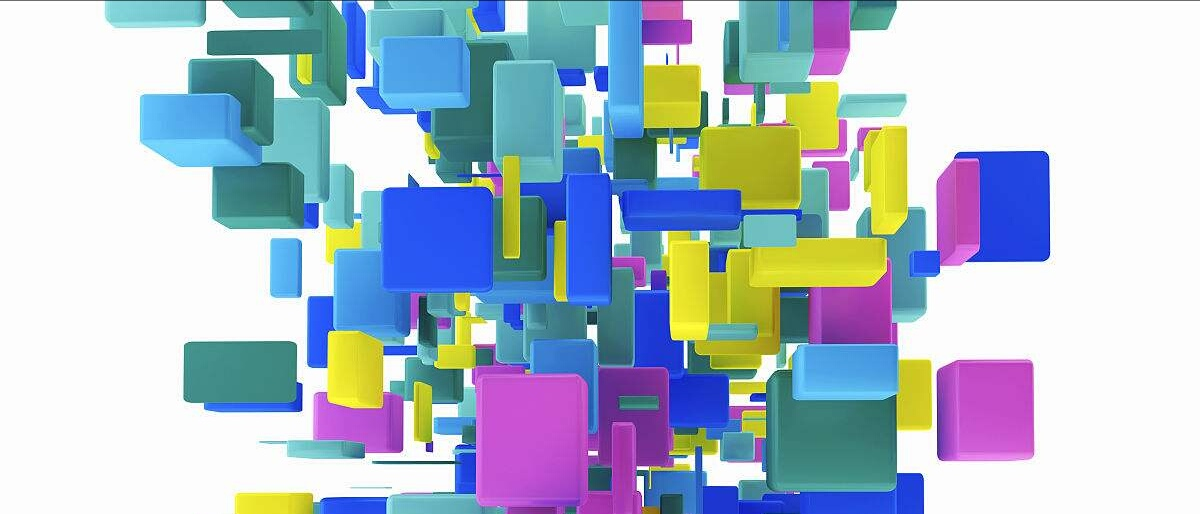1. 前言

作为无监督聚类算法中的代表——K均值聚类（Kmeans）算法，该算法的主要作用是将相似的样本自动归到一个类别中。所谓的监督算法，就是输入样本没有对应的输出或标签。聚类（clustering）试图将数据集中的样本划分为若干个通常是不相交的子集，每个子集称为一个“簇（cluster）”，聚类既能作为一个单独过程，用于找寻数据内在的分布结构，也可作为分类等其他学习任务的前去过程。——《Machine Learning》

聚类算法也许是机器学习中“新算法”出现最多、最快的领域，一个重要的原因是聚类不存在客观标准，给定数据集总能从某个角度找到以往算法未覆盖的某种标准从而设计出新算法。Kmeans算法十分简单易懂而且非常有效，但是合理的确定K值和K个初始类簇中心点对于聚类效果的好坏有很大的影响。众多的论文基于此都提出了各自行之有效的解决方案，新的改进算法仍然不断被提出，此类文章大家可以在Web Of Science中搜索。
尽管Kmeans算法在MATLAB、Python等语言的工具箱函数中都有自带的函数可供调用，但作为机器学习的研究者新来说要设计出新的算法，有时就得“定制”自己的Kmeans函数了。自己动手编写无疑也更加能理解算法的具体过程，接下来就让我们进入正题吧

2. Kmeans算法的原理与理解
Kmeans算法是最常用的聚类算法，主要思想是:在给定K值和K个初始类簇中心点的情况下，把每个点(亦即数据记录)分到离其最近的类簇中心点所代表的类簇中，所有点分配完毕之后，根据一个类簇内的所有点重新计算该类簇的中心点(取平均值)，然后再迭代的进行分配点和更新类簇中心点的步骤，直至类簇中心点的变化很小，或者达到指定的迭代次数。
2.1 基本原理
假定给定数据样本X，包含了n个对象$X=\left \{ X_{1} ,X_{2},X_{3},...,X_{n}\right \}$,其中每个对象都具有m个维度的属性。Kmeans算法的目标是将n个对象依据对象间的相似性聚集到指定的k个类簇中，每个对象属于且仅属于一个其到类簇中心距离最小的类簇中。对于Kmeans，首先需要初始化k个聚类中心$\left \{ C_{1} ,C_{2},C_{3},...,C_{k}\right \},1,然后通过计算每一个对象到每一个聚类中心的欧式距离，如下式所示$dis(X_{i},C_{j})=\sqrt{\sum_{t=1}^{m}(X_{it}-C_{jt})^{2}}$
上式中，$X_{i}$表示第i个对象$1\leq i\leq n$,$C_{j}$表示第j个聚类中心的$1\leq j\leq k$，$X_{it}$表示第i个对象的第t个属性，$1\leq t\leq m$，$C_{jt}$表示第j个聚类中心的第t个属性。
依次比较每一个对象到每一个聚类中心的距离，将对象分配到距离最近的聚类中心的类簇中，得到k个类簇$\left \{ S_{1},S_{2},S_{3},...,S_{k} \right \}$

Kmeans算法用中心定义了类簇的原型，类簇中心就是类簇内所有对象在各个维度的均值，其计算公式如下$C_{t}=\frac{\sum_{X_{i}\in S_{l}}X_{i}}{\left | S_{l} \right |}$
式中，$C_{l}$表示第l个聚类的中心，$1\leq l\leq k$，$\left | S_{l} \right |$表示第l个类簇中对象的个数，$X_{i}$表示第l个类簇中第i个对象，$1\leq i\leq\left | S_{l} \right |$。
2.2 算法流程

输入：样本集$D=\left \{ x_{1},x_{2},x_{3},...,x_{m} \right \}$；聚类簇数k.
过程：
1：从D中随机选择k个样本作为初始均值向量$\left \{ \mu _{1},\mu _{2},\mu _{3},...,\mu _{k} \right \}$
2：repeat
3：      令$C_{i}=\varnothing (1\leqslant i\leqslant k)$
4：     for j=1,2,…,m  do
5：           计算样本$x_{j}$与各均值向量$\mu_{i}(1\leqslant i\leqslant k)$的距离：$d_{ji}=\left \| x_{j}-\mu_{i} \right \|_{2}$;
6：            根据距离最近的均值向量确定$x_{j}$的簇标记：$\lambda _{j}=arg min_{i\in \left \{ 1,2,3,...,k \right \}}d_{ji}$；
7：            将样本$x_{j}$划入相应的簇：$C_{\lambda_{j}}=C_{\lambda_{j}}\cup \left \{ x_{j} \right \}；$
8：        end for
9：        for i=1,2,…,k do
10:               计算新均值向量：$\mu_{i}^{'}=\frac{1}{\left | C_{i} \right |}\sum _{x\in C_{i}}x$;
11：             if  $\mu_{i}^{'}\neq \mu_{i}$  then
12：                 将当前均值向量 $\mu_{i}$更新为$\mu_{i}^{'}$
13：             else
14：                 保持当前均值不变
15：                 end if
16：                 end for
17：until  当前均值向量均未更新
输出：簇划分$C=\left \{ C_{1} ,C_{2},...,C_{k} \right \}$

以上算法流程引自周志华《机器学习》，从流程来看K-means算法计算步骤基本上可以概括为两个部分：（1）计算每一个对象到类簇中心的距离；（2）根据类簇内的对象计算新的簇类中心。

3. 编程实现
为了方便应用我们将其编写为一个M函数KMeans()，首先需要确定函数的输入输出。这里输入参数为：data，K，iniCentriods，iterations（其中data为输入的不带标号的数据集数据矩阵，大小为numOfDatanumOfAttributes，K为数据分的类簇数目，iniCentriods为自行指定的初始聚类中心矩阵，大小为KnumOfAttributes，iterations为算法迭代次数。）
输出参数为：Idx，centroids，Distance（Idx为返回的分类标号， centroids为每一类的中心，Distance为类内总距离）
根据前面2.2节中的算法流程编写Kmeans算法的MATLAB程序如下
%% Kmeans算法
% 输入：
% data 输入的不带分类标号的数据
% K 数据一共分多少类
% iniCentriods 自行指定初始聚类中心
% iterations 迭代次数

% 输出：
% Idx 返回的分类标号
% centroids 每一类的中心
% Distance 类内总距离

function [Idx,centroids,Distance]=KMeans(data,K,iniCentriods,iterations)
[numOfData,numOfAttr]=size(data); % numOfData是数据个数，numOfAttr是数据维数
centroids=iniCentriods;
%% 迭代
for iter=1:iterations
pre_centroids=centroids;% 上一次求得的中心位置

tags=zeros(numOfData,K);
%% 寻找最近中心，更新中心
for i=1:numOfData
D=zeros(1,K);% 每个数据点与每个聚类中心的标准差
Dist=D;

% 计算每个点到每个中心点的标准差
for j=1:K
Dist(j)=norm(data(i,:)-centroids(j,:),2);
end

[minDistance,index]=min(Dist);% 寻找距离最小的类别索引
tags(i,index)=1;% 标记最小距离所处的位置（类别）
end

%% 取均值更新聚类中心点
for i=1:K
if sum(tags(:,i))~=0
% 未出现空类，计算均值作为下一聚类中心
for j=1:numOfAttr
centroids(i,j)=sum(tags(:,i).*data(:,j))/sum(tags(:,i));
end
else % 如果出现空类，从数据集中随机选中一个点作为中心
randidx = randperm(size(data, 1));
centroids(i,:) = data(randidx(1),:);
tags(randidx,:)=0;
tags(randidx,i)=1;
end
end

if sum(norm(pre_centroids-centroids,2))<0.001  % 不断迭代直到位置不再变化
break;
end

end

%% 计算输出结果
Distance=zeros(numOfData,1);
Idx=zeros(numOfData,1);
for i=1:numOfData
D=zeros(1,K);% 每个数据点与每个聚类中心的标准差
Dist=D;
% 计算每个点到每个中心点的标准差
for j=1:K
Dist(j)=norm(data(i,:)-centroids(j,:),2);
end

[distance,idx]=min(Dist);% 寻找距离最小的类别索引
distance=Dist(idx);

Distance(i)=distance;
Idx(i)=idx;
end
Distance=sum(Distance,1);% 计算类内总距离
end

在以上代码中其最主要部分在于第18至58行，进行寻找最近中心和求取均值更新聚类中心点。值得注意的是，在聚类过程中可能会出现空类即代码第44行那样，为保证算法的继续运行，从数据集中随机选取一个点作为中心。
4. 聚类结果评价
为了验证编写的Kmeans函数的性能，这里对想用的UCI数据集Iris数据集进行聚类并计算聚类的准确率，Iris数据集可以在http://archive.ics.uci.edu/ml/index.php上下载得到。首先读取Iris数据集，自行指定初始聚类中心调用前面编写的KMeans函数进行聚类，然后计算聚类的准确率，其代码如下
clear
data=data(:,2:end);

matrix=[5.9016,2.7484,4.3935,1.4339;6.8500,3.0737,5.7421,2.0711;5.0060,3.4280,1.4620,0.2460];
[Idx,C,distance]=KMeans(data,3,matrix,500);
Distance=sum(distance)

c1=Idx(1:50,1);c2=Idx(51:100,1);c3=Idx(101:150,1);
accuracy=(sum(c1==mode(Idx(1:50,1)))+sum(c2==mode(Idx(51:100,1)))+sum(c3==mode(Idx(101:150,1))))/150

为方便使用Iris数据集经过了一些整理，这里将最后一列的带字符串的标签Iris-setosa，Iris-versicolor，Iris-virginica分别用数字1,2,3代替并移到了第一列，所以第三行选取的是从第二列至最后一列的数据。第5行中的matrix是查阅论文得到的一个初始聚类中心，正好用来比对聚类结果。第6行则调用KMeans()函数进行聚类，得到聚类标号和类内距离。对每类的类内距离求和即得到总的距离Distance，如第7行。准确率的计算有点麻烦，因为不能直接用KMeans计算后得到的标号跟原数据集中的标号对比计算准确率，KMeans只需要也只能将那些“相似”的数据点聚集到一类中，而给这一类数据的标号却是可能跟原数据集不同的。
这里采用一个简单的方法，从原数据集的标签可以看出第1-50个数据点为一类（Iris-setosa），第51-100为一类（Iris-versicolor），第101-150为一类（Iris-virginica），因此只需确定每50个数据点中的聚类标号是不是一致。取它们之中数目最多的标号作为正确的个数，最终比上数据集的总数即为准确率。以上代码运行结果如下所示。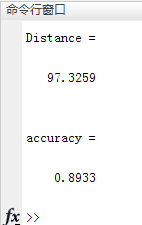5. 类簇中心点的选取
KMeans算法本身思想比较简单，但是合理的确定K值和K个初始类簇中心点对于聚类效果的好坏有很大的影响。最简单的确定初始类簇中心点的方法是随机产生数据大小范围内的K个点作为初始的簇类中心点。随机产生初始点并进行测试的程序代码如下
clear
data=data(:,2:end);
K=3;

%% 产生随机初始点
[numOfData,numOfAttr]=size(data);   % numOfData是数据个数，numOfAttr是数据维数

centroids=zeros(K,numOfAttr);       % 随机初始化，最终迭代到每一类的中心位置
maxAttr=zeros(numOfAttr);        % 每一维最大的数
minAttr=zeros(numOfAttr);        % 每一维最小的数
for i=1:numOfAttr
maxAttr(i)=max(data(:,i));    % 每一维最大的数
minAttr(i)=min(data(:,i));    % 每一维最小的数
for j=1:K
centroids(j,i)=maxAttr(i)+(minAttr(i)-maxAttr(i))*rand();  % 随机初始化，选取每一维[min max]中初始化
end
end

[Idx,C,distance]=KMeans(data,K,centroids,500);% 调用KMeans
Distance=sum(distance)% 计算类内距离之和

%% 计算准确率
c1=Idx(1:50,1);c2=Idx(51:100,1);c3=Idx(101:150,1);
Accuracy=(sum(c1==mode(Idx(1:50,1)))+sum(c2==mode(Idx(51:100,1)))+sum(c3==mode(Idx(101:150,1))))/numOfData

可以多运行几次以上代码，可以看出由于初始点事随机选取的每次运行得到的结果有所差异。这也是基本Kmeans算法的一个缺点，随着众多改进算法的提出Kmeans算法的这一问题也得到改善，深入了解的朋友可以查阅相关论文。
6. 结束语
本博文的完整MATLAB程序文件与整理好的数据集文件已经上传，下载即可运行。下载地址如下
点击下载：本文Kmeans算法M函数及测试完整文件
【公众号获取】
本人微信公众号已创建，扫描以下二维码并关注公众号“AI技术研究与分享”，后台回复“KM20180516”即可获取全部资源文件信息。由于编者能力有限，代码即使经过了多次校对，也难免会有疏漏之处。希望您能热心指出其中的错误，以便下次修改时能以一个更完美更严谨的样子，呈现在大家面前。同时如果有更好的实现方法也请您不吝赐教。


展开全文人工智能
• K-means算法的主要思想就是以空间中的K个点为中心进行聚类，对最靠近它的对象进行归类。通过迭代的方法不断的更新各聚类中心的值，直到最好的聚类结果。 主要步骤： 在N个数据中，随机挑选K个数据（也就是最后...
K-means算法的主要思想就是以空间中的K个点为中心进行聚类，对最靠近它的对象进行归类。通过迭代的方法不断的更新各聚类中心的值，直到最好的聚类结果。

K的取值：
确定聚类数K没有最佳的方法，通常需要根据具体的问题由人工进行选择。非监督聚类没有比较直接的聚类评估方法，但是可以从簇内的稠密程度和簇间的离散程度来评估聚类的效果。最常见的方法有轮廓系数Silhouette Coefficient和Calinski-Harabaz Index。其中Calinski-Harabaz Index计算直接简单，得到的结果越大则聚类效果越好。计算公式如下：
$s(K)=(\frac{tr(B_{k})}{tr(W_{k})}\cdot \frac{m-k}{k-1})$
其中：m为训练集样本数，k为类别数。Bk为类别之间的协方差矩阵，Wk为内部数据之间的协方差矩阵。tr为矩阵的迹。
也就是说内部数据的协方差越小越好，类别之间的协方差越大越好，这样对应的Calinski-Harabaz Index分数也就越高

主要步骤：

在N个数据中，随机挑选K个数据（也就是最后聚类为K类）做为聚类的初始中心。
分别计算每个数据点到这K个中心点的欧式距离，离哪个中心点最近就分配到哪个簇中。
重新计算这K个簇数据的坐标均值，将新的均值作为聚类的中心。
重复2和3步骤，直到簇中心的坐标不再变换或者达到规定的迭代次数，形成最终的K个聚类。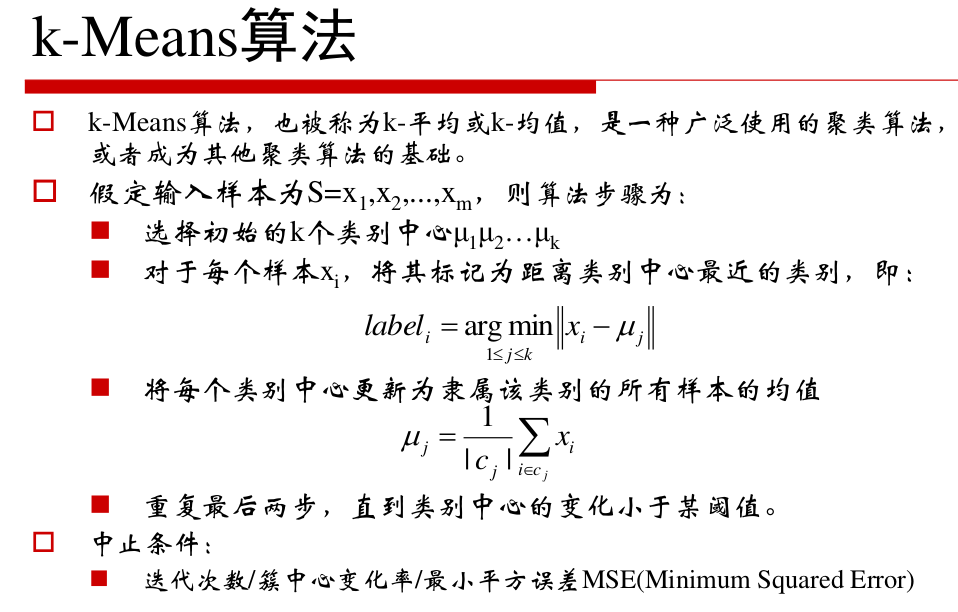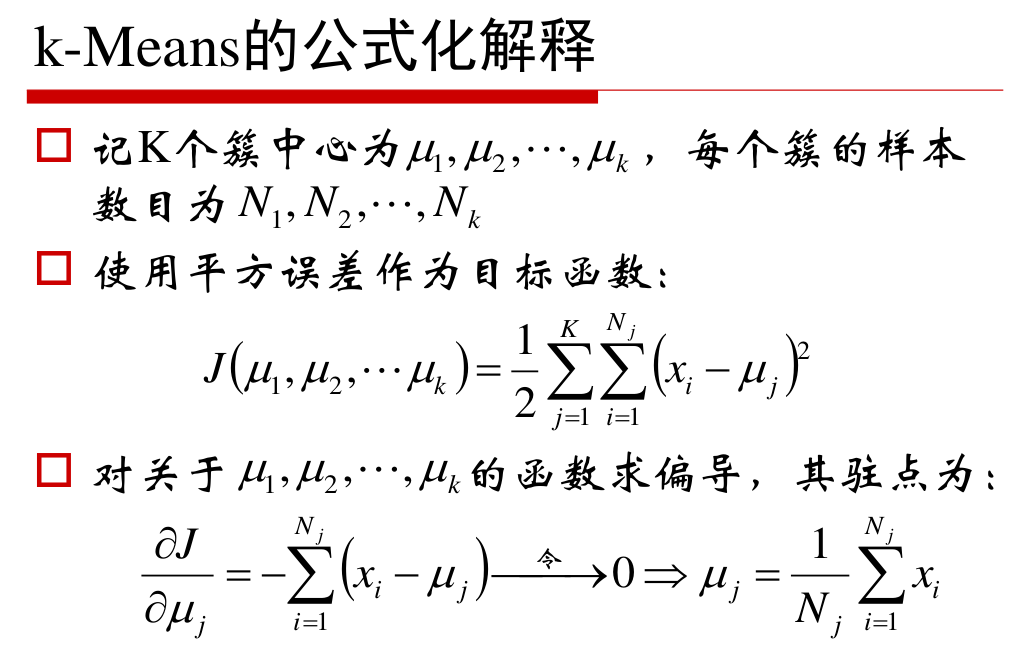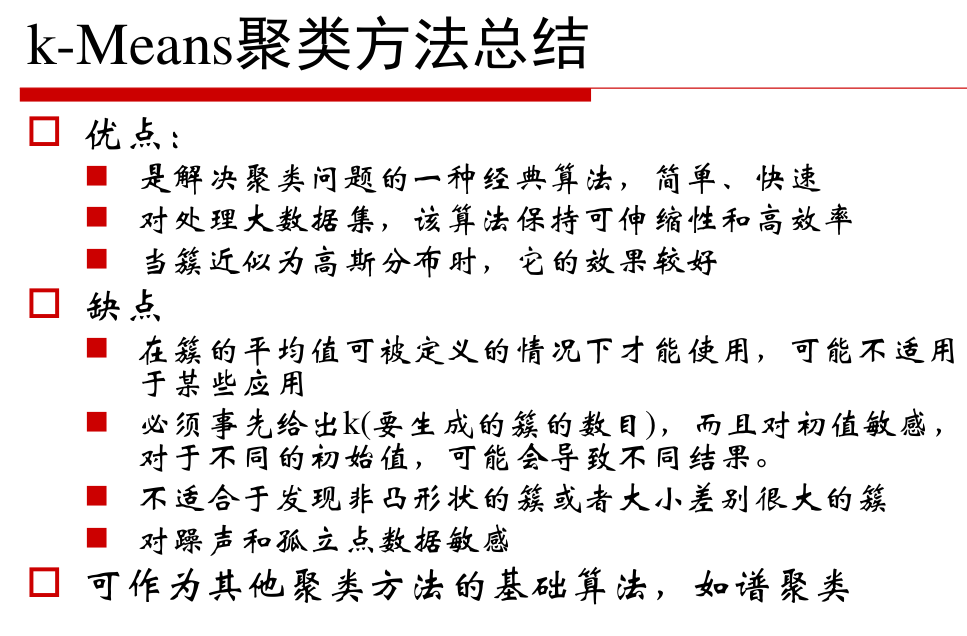源码实现：
有两个py文件。kmeans.py 和 kmeanstest.py。源码在kmeans.py中，kmeanstest.py为实例（后期会给出sklean运行代码）。
# %load kmeans.py

#导入模块
import numpy as np
import matplotlib.pyplot as plt
from math import sqrt

#计算欧式距离
def eucDistance(vec1,vec2):
return sqrt(sum(pow(vec2-vec1,2)))

#初始聚类中心选择
def initCentroids(dataSet,k):
numSamples,dim = dataSet.shape
centroids = np.zeros((k,dim))
for i in range(k):
index = int(np.random.uniform(0,numSamples))
centroids[i,:] = dataSet[index,:]
return centroids

#K-means聚类算法，迭代
def kmeanss(dataSet,k):
numSamples = dataSet.shape
clusterAssement = np.mat(np.zeros((numSamples,2)))
clusterChanged = True
#  初始化聚类中心
centroids = initCentroids(dataSet,k)
while clusterChanged:
clusterChanged = False
for i in range(numSamples):
minDist = 100000.0
minIndex = 0
# 找到哪个与哪个中心最近
for j in range(k):
distance = eucDistance(centroids[j,:],dataSet[i,:])
if distance<minDist:
minDist = distance
minIndex = j
# 更新簇
clusterAssement[i,:] = minIndex,minDist**2
if clusterAssement[i,0]!=minIndex:
clusterChanged = True
# 坐标均值更新簇中心
for j in range(k):
pointsInCluster = dataSet[np.nonzero(clusterAssement[:0].A==j)]
centroids[j,:] = np.mean(pointsInCluster,axis=0)
print('Congratulations,cluster complete!')
return centroids,clusterAssement

#聚类结果显示
def showCluster(dataSet,k,centroids,clusterAssement):
numSamples,dim = dataSet.shape
mark = ['or','ob','og','ok','^r','+r','<r','pr']
if k>len(mark):
print('Sorry!')
return 1
for i in np.xrange(numSamples):
markIndex = int(clusterAssement[i,0])
plt.plot(centroids[i,0],centroids[i,1],mark[i],markersize=12)
plt.show()

有如下80个二维数据的样本，存在testSet的文档中，经过数据预处理和分析得知数据集共有4个类，所以确定K=4。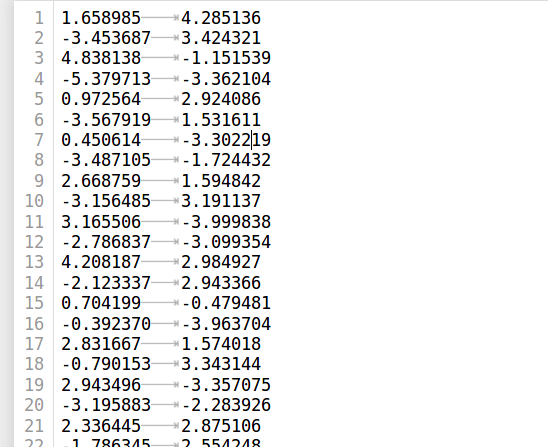# %load kmeanstest.py

#导入模块
import kmeans
import  numpy as np
import matplotlib.pyplot as plt
from math import sqrt

#从文件加载数据集
dataSet=[]
fileIn = open('./testSet.txt')
lineArr = line.strip().split('\t')
dataSet.append([float(lineArr),float(lineArr)])

#调用k-means进行数据聚类
dataSet = np.mat(dataSet)
k = 4
centroids,clusterAssement = kmeans.kmeanss(dataSet,k)

#显示结果
kmeans.showCluster(dataSet,centroids,clusterAssement)

使用k-means算法聚类结果如下：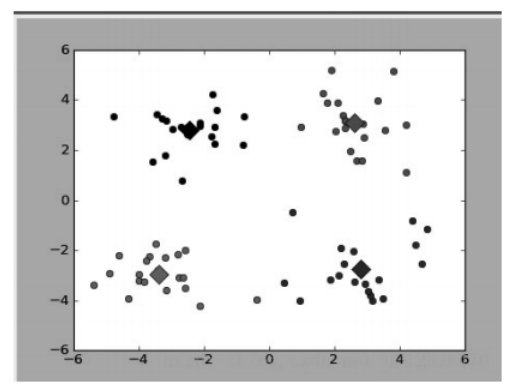用sklearn实现Kmeans：
随机生成二维多类数据，样本大致分为4类，调用sklearn中的聚类函数进行分析不同k值对聚类结果的影响以及评估方法。
import numpy as np
import matplotlib.pyplot as plt
from sklearn.datasets.samples_generator import make_blobs
from sklearn.cluster import KMeans

X, y = make_blobs(n_samples=1000, n_features=2, centers=[[-1,-1], [0,0], [1,1], [2,2]],\
cluster_std = [0.4, 0.2, 0.2, 0.2], random_state=9)
plt.scatter(X[:, 0], X[:, 1], marker='+')
plt.show()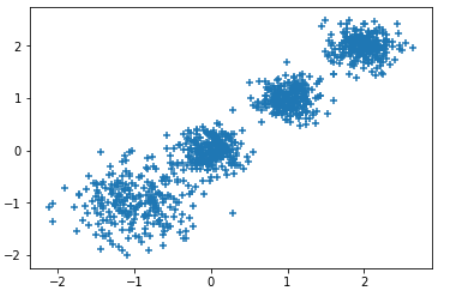K=2：
y_pred = KMeans(n_clusters=2, random_state=9).fit_predict(X)
plt.scatter(X[:, 0], X[:, 1], c=y_pred)
plt.show()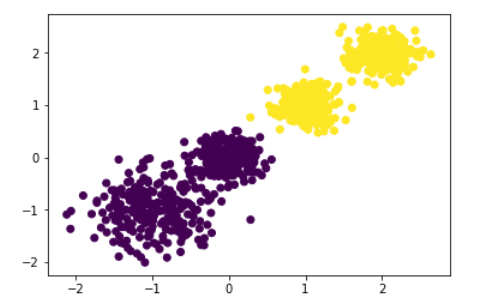调用Calinski-Harabaz Index来评估聚类分数:
from sklearn import metrics
metrics.calinski_harabaz_score(X, y_pred)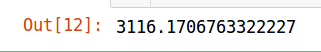K=3:
y_pred = KMeans(n_clusters=3, random_state=9).fit_predict(X)
plt.scatter(X[:, 0], X[:, 1], c=y_pred)
plt.show()
from sklearn import metrics
metrics.calinski_harabaz_score(X, y_pred)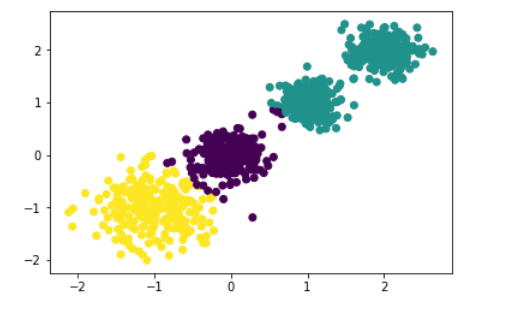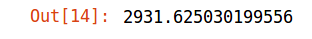K=4:
y_pred = KMeans(n_clusters=4, random_state=9).fit_predict(X)
plt.scatter(X[:, 0], X[:, 1], c=y_pred)
plt.show()
from sklearn import metrics
metrics.calinski_harabaz_score(X, y_pred)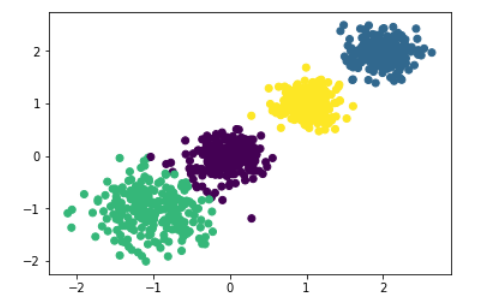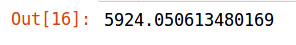可以发现当K=4的时候得分最高，在往后分值就会有所下降，所以现在的K值比较合适。
K=5:
y_pred = KMeans(n_clusters=5, random_state=9).fit_predict(X)
plt.scatter(X[:, 0], X[:, 1], c=y_pred)
plt.show()
from sklearn import metrics
metrics.calinski_harabaz_score(X, y_pred)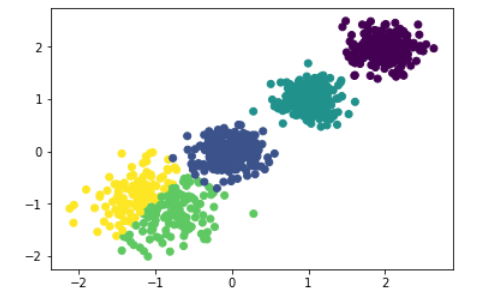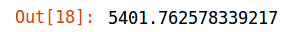展开全文机器学习 聚类
• ## 5 分钟带你弄懂 k-means 聚类

万次阅读 多人点赞 2017-11-08 16:16:23
聚类与分类的区别分类：类别是已知的，通过对已知分类的数据进行训练和学习，找到这些不同类的特征，再对未分类的数据进行分类。属于监督学习。聚类：事先不知道数据会分为几类，通过聚类分析将数据聚合成几个群体。...
聚类与分类的区别

分类：类别是已知的，通过对已知分类的数据进行训练和学习，找到这些不同类的特征，再对未分类的数据进行分类。属于监督学习。

聚类：事先不知道数据会分为几类，通过聚类分析将数据聚合成几个群体。聚类不需要对数据进行训练和学习。属于无监督学习。

关于监督学习和无监督学习，这里给一个简单的介绍：是否有监督，就看输入数据是否有标签，输入数据有标签，则为有监督学习，否则为无监督学习。更详尽的解释会在后续博文更新，这里不细说。

k-means 聚类

聚类算法有很多种，K-Means 是聚类算法中的最常用的一种，算法最大的特点是简单，好理解，运算速度快，但是只能应用于连续型的数据，并且一定要在聚类前需要手工指定要分成几类。

K-Means 聚类算法的大致意思就是“物以类聚，人以群分”：

首先输入 k 的值，即我们指定希望通过聚类得到 k 个分组；
从数据集中随机选取 k 个数据点作为初始大佬（质心）；
对集合中每一个小弟，计算与每一个大佬的距离，离哪个大佬距离近，就跟定哪个大佬。
这时每一个大佬手下都聚集了一票小弟，这时候召开选举大会，每一群选出新的大佬（即通过算法选出新的质心）。
如果新大佬和老大佬之间的距离小于某一个设置的阈值（表示重新计算的质心的位置变化不大，趋于稳定，或者说收敛），可以认为我们进行的聚类已经达到期望的结果，算法终止。
如果新大佬和老大佬距离变化很大，需要迭代3~5步骤。
说了这么多，估计还是有点糊涂，下面举个非常形象简单的例子：
有6个点，从图上看应该可以分成两堆，前三个点一堆，后三个点另一堆。现在我手工地把 k-means 计算过程演示一下，同时检验是不是和预期一致：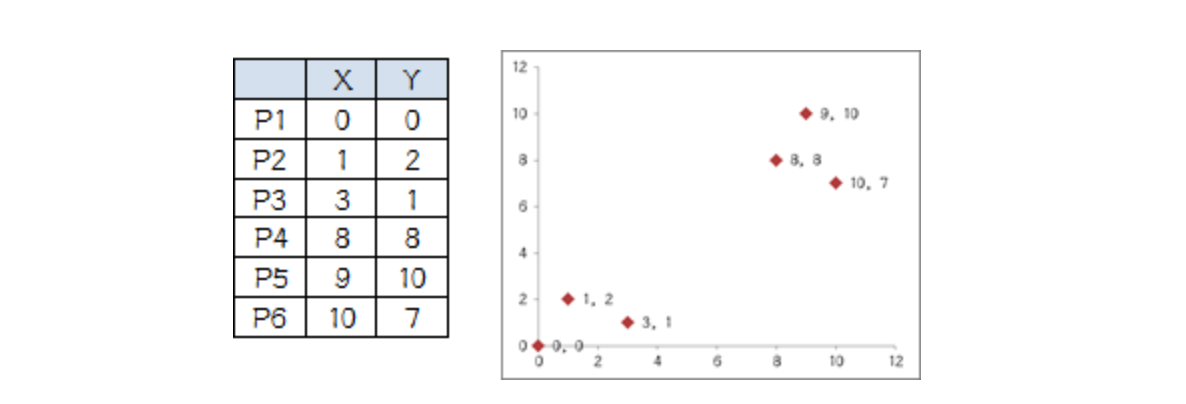1.设定 k 值为2

2.选择初始大佬（就选 P1 和 P2）

3.计算小弟与大佬的距离：从上图可以看出，所有的小弟都离 P2 更近，所以次站队的结果是：

A 组：P1
B 组：P2、P3、P4、P5、P6

4.召开选举大会：

A 组没什么可选的，大佬就是自己
B 组有5个人，需要重新选大佬，这里要注意选大佬的方法是每个人 X 坐标的平均值和 Y 坐标的平均值组成的新的点，为新大佬，也就是说这个大佬是“虚拟的”。因此，B 组选出新大哥的坐标为：P 哥（（1+3+8+9+10）/5，（2+1+8+10+7）/5）=（6.2，5.6）。
综合两组，新大哥为 P1（0，0），P哥（6.2，5.6），而P2-P6重新成为小弟。

5.再次计算小弟到大佬的距离：这时可以看到P2、P3离P1更近，P4、P5、P6离P哥更近，所以第二次站队的结果是：

A 组：P1、P2、P3
B 组：P4、P5、P6（虚拟大哥这时候消失）

6.第二届选举大会：
同样的方法选出新的虚拟大佬：P哥1（1.33，1），P哥2（9，8.33），P1-P6都成为小弟。

7.第三次计算小弟到大佬的距离：这时可以看到 P1、P2、P3 离 P哥1 更近，P4、P5、P6离 P哥2 更近，所以第二次站队的结果是：
A 组：P1、P2、P3
B 组：P4、P5、P6

我们可以发现，这次站队的结果和上次没有任何变化了，说明已经收敛，聚类结束，聚类结果和我们最开始设想的结果完全一致。

K-Means 聚类 MATLAB 实现

关于 K-Means 的算法具体代码，网上有各种版本，这里也不赘述了，下面结合 MATLAB 中的一些函数给出一个较为简洁的版本：

X2 = zscore(X);      % zscore方法标准化数据
Y2 = pdist(X2);      % 计算距离（默认欧式距离）
T = cluster(Z2,6);   % 创建聚类
H = dendrogram(Z2);  %作出系谱图

最终聚类系谱图如下所示：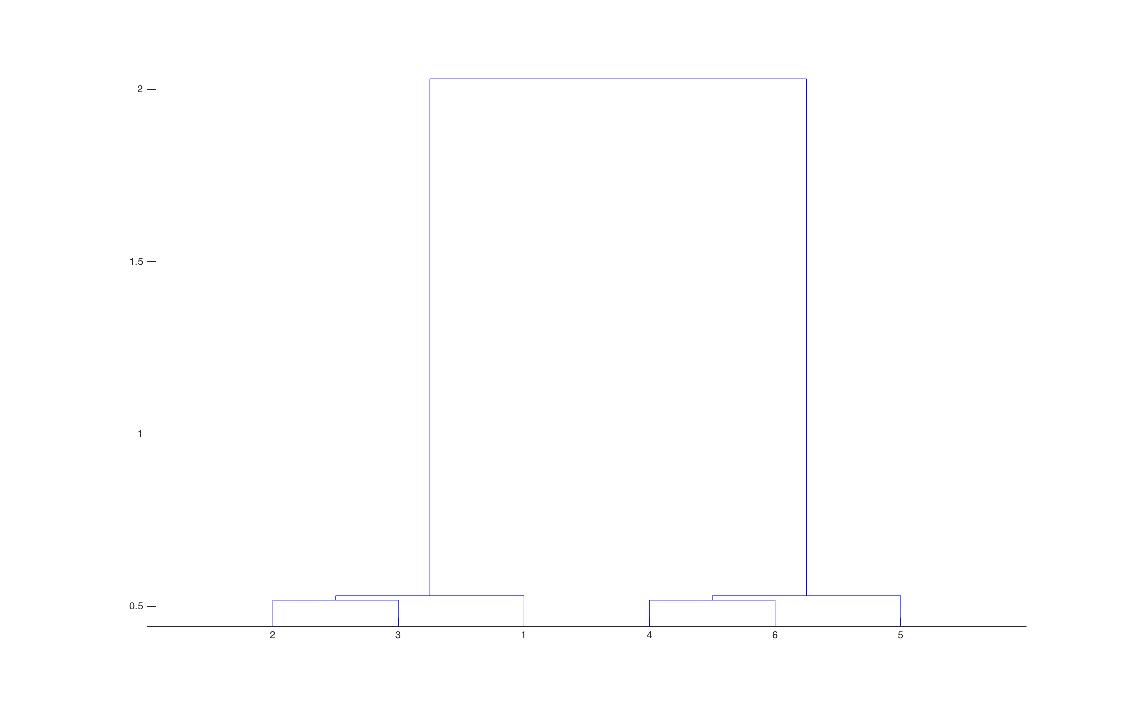当然，MATLAB 也提供了 kmeans() 函数可供直接聚类使用，详情可参与其文档。
展开全文聚类 分类 k-means 无监督学习 MATLAB
• ## K-means原理、优化及应用

万次阅读 多人点赞 2018-08-23 14:48:59
K-Means算法是无监督的聚类算法，它实现起来比较简单，聚类效果也不错，因此应用很广泛。K-Means算法有大量的变体，本文就从最传统的K-Means算法讲起，在其基础上讲述K-Means的优化变体方法。包括初始化优化K-Means+...
　K-Means算法是无监督的聚类算法，它实现起来比较简单，聚类效果也不错，因此应用很广泛。K-Means算法有大量的变体，本文就从最传统的K-Means算法讲起，在其基础上讲述K-Means的优化变体方法。包括初始化优化K-Means++, 距离计算优化elkan K-Means算法和大数据情况下的优化Mini Batch K-Means算法。

1. K-Means原理初探

K-Means算法的思想很简单，对于给定的样本集，按照样本之间的距离大小，将样本集划分为K个簇。让簇内的点尽量紧密的连在一起，而让簇间的距离尽量的大。

如果用数据表达式表示，假设簇划分为(C1,C2,...Ck)(C1,C2,...Ck)，则我们的目标是最小化平方误差E：

E=∑i=1k∑x∈Ci||x−μi||22E=∑i=1k∑x∈Ci||x−μi||22

其中μiμi是簇CiCi的均值向量，有时也称为质心，表达式为：

μi=1|Ci|∑x∈Cixμi=1|Ci|∑x∈Cix

如果我们想直接求上式的最小值并不容易，这是一个NP难的问题，因此只能采用启发式的迭代方法。

K-Means采用的启发式方式很简单，用下面一组图就可以形象的描述。上图a表达了初始的数据集，假设k=2。在图b中，我们随机选择了两个k类所对应的类别质心，即图中的红色质心和蓝色质心，然后分别求样本中所有点到这两个质心的距离，并标记每个样本的类别为和该样本距离最小的质心的类别，如图c所示，经过计算样本和红色质心和蓝色质心的距离，我们得到了所有样本点的第一轮迭代后的类别。此时我们对我们当前标记为红色和蓝色的点分别求其新的质心，如图4所示，新的红色质心和蓝色质心的位置已经发生了变动。图e和图f重复了我们在图c和图d的过程，即将所有点的类别标记为距离最近的质心的类别并求新的质心。最终我们得到的两个类别如图f。

当然在实际K-Mean算法中，我们一般会多次运行图c和图d，才能达到最终的比较优的类别。

2. 传统K-Means算法流程

算法步骤：

1.（随机）选择K个聚类的初始中心；

2.对任意一个样本点，求其到K个聚类中心的距离，将样本点归类到距离最小的中心的聚类，如此迭代n次；

3.每次迭代过程中，利用均值等方法更新各个聚类的中心点(质心)；

4.对K个聚类中心，利用2,3步迭代更新后，如果位置点变化很小(可以设置阈值)，则认为达到稳定状态，迭代结束，对不同的聚类块和聚类中心可选择不同的颜色标注。

优点
1）原理比较简单，实现也是很容易，收敛速度快。
2）聚类效果较优。
3）算法的可解释度比较强。
4）主要需要调参的参数仅仅是簇数k。

缺点
1）K值的选取不好把握
2）对于不是凸的数据集比较难收敛
3）如果各隐含类别的数据不平衡，比如各隐含类别的数据量严重失衡，或者各隐含类别的方差不同，则聚类效果不佳。
4） 最终结果和初始点的选择有关，容易陷入局部最优。
5） 对噪音和异常点比较的敏感。

3. K-Means初始化优化K-Means++

k个初始化的质心的位置选择对最后的聚类结果和运行时间都有很大的影响，因此需要选择合适的k个质心。如果仅仅是完全随机的选择，有可能导致算法收敛很慢。K-Means++算法就是对K-Means随机初始化质心的方法的优化。

K-Means++的对于初始化质心的优化策略也很简单，如下：

a)  从输入的数据点集合中随机选择一个点作为第一个聚类中心μ1
b) 对于数据集中的每一个点xi，计算它与已选择的聚类中心中最近聚类中心的距离D(xi)=argmin||xi−μr||^2……r=1,2,...kselected

c) 选择下一个新的数据点作为新的聚类中心，选择的原则是：D(x)较大的点，被选取作为聚类中心的概率较大
d) 重复b和c直到选择出k个聚类质心
e) 利用这k个质心来作为初始化质心去运行标准的K-Means算法

K-Means++对初始聚类中心点的选取做了优化，简要来说就是使初始聚类中心点尽可能的分散开来，这样可以有效的减少迭代次数，加快运算速度。

4. K-Means距离计算优化elkan K-Means

在传统的K-Means算法中，我们在每轮迭代时，要计算所有的样本点到所有的质心的距离，这样会比较的耗时。那么，对于距离的计算有没有能够简化的地方呢？elkan K-Means算法就是从这块入手加以改进。它的目标是减少不必要的距离的计算。那么哪些距离不需要计算呢？

elkan K-Means利用了两边之和大于等于第三边,以及两边之差小于第三边的三角形性质，来减少距离的计算。

第一种规律是对于一个样本点xx和两个质心μj1,μj2。如果我们预先计算出了这两个质心之间的距离D(j1,j2)，则如果计算发现2D(x,j1)≤D(j1,j2),我们立即就可以知道D(x,j1)≤D(x,j2)。此时我们不需要再计算D(x,j2),也就是说省了一步距离计算。

第二种规律是对于一个样本点x和两个质心μj1,μj2。我们可以得到D(x,j2)≥max{0,D(x,j1)−D(j1,j2)}。这个从三角形的性质也很容易得到。

利用上边的两个规律，elkan K-Means比起传统的K-Means迭代速度有很大的提高。但是如果我们的样本的特征是稀疏的，有缺失值的话，这个方法就不使用了，此时某些距离无法计算，则不能使用该算法。

个人理解，第一个规律简单有效，很容易理解，但是第二个规律对简化运算量的意义不是很大。

5. 大样本优化Mini Batch K-Means

在统的K-Means算法中，要计算所有的样本点到所有的质心的距离。如果样本量非常大，比如达到10万以上，特征有100以上，此时用传统的K-Means算法非常的耗时，就算加上elkan K-Means优化也依旧。在大数据时代，这样的场景越来越多。此时Mini Batch K-Means应运而生。

顾名思义，Mini Batch，也就是用样本集中的一部分的样本来做传统的K-Means，这样可以避免样本量太大时的计算难题，算法收敛速度大大加快。当然此时的代价就是我们的聚类的精确度也会有一些降低。一般来说这个降低的幅度在可以接受的范围之内。

在Mini Batch K-Means中，我们会选择一个合适的批样本大小batch size，我们仅仅用batch size个样本来做K-Means聚类。那么这batch size个样本怎么来的？一般是通过无放回的随机采样得到的。

为了增加算法的准确性，我们一般会多跑几次Mini Batch K-Means算法，用得到不同的随机采样集来得到聚类簇，选择其中最优的聚类簇。

该算法的迭代步骤有两步：

1：从数据集中随机抽取一些数据形成小批量，把他们分配给最近的质心

2：更新质心

与K均值算法相比，数据的更新是在每一个小的样本集上。对于每一个小批量，通过计算平均值得到更新质心，并把小批量里的数据分配给该质心，随着迭代次数的增加，这些质心的变化是逐渐减小的，直到质心稳定或者达到指定的迭代次数，停止计算

Mini Batch K-Means比K-Means有更快的 收敛速度，但同时也降低了聚类的效果，但是在实际项目中却表现得不明显。个人理解，Mini Batch K-Means通过小样本的实验，可以初步预测聚类中心的位置，对n次Mini Batch K-Means的聚类结果取均值作为大样本实验初始聚类中心的位置，也能够减少运算量，加快聚类速度。

下边给出显示上边这副图的代码，也是对K-Means和Mini Batch K-Means算法的一个比较：

import time

import numpy as np
import matplotlib.pyplot as plt

from sklearn.cluster import MiniBatchKMeans, KMeans
from sklearn.metrics.pairwise import pairwise_distances_argmin
from sklearn.datasets.samples_generator import make_blobs

##############################################################################
# Generate sample data
np.random.seed(0)

batch_size = 45
centers = [[1, 1], [-1, -1], [1, -1]] #初始化三个中心
n_clusters = len(centers)       #聚类的数目为3
#产生3000组两维的数据，以上边三个点为中心，以（-10,10）为边界，数据集的标准差是0.7
X, labels_true = make_blobs(n_samples=3000, centers=centers, cluster_std=0.7)

##############################################################################
# Compute clustering with Means

k_means = KMeans(init='k-means++', n_clusters=3, n_init=10)
t0 = time.time() #当前时间
k_means.fit(X)
#使用K-Means 对 3000数据集训练算法的时间消耗
t_batch = time.time() - t0

##############################################################################
# Compute clustering with MiniBatchKMeans

mbk = MiniBatchKMeans(init='k-means++', n_clusters=3, batch_size=batch_size,
n_init=10, max_no_improvement=10, verbose=0)
t0 = time.time()
mbk.fit(X)
#使用MiniBatchKMeans 对 3000数据集训练算法的时间消耗
t_mini_batch = time.time() - t0

##############################################################################
# Plot result

#创建一个绘图对象, 并设置对象的宽度和高度, 如果不创建直接调用plot, Matplotlib会直接创建一个绘图对象
'''
当绘图对象中有多个轴的时候，可以通过工具栏中的Configure Subplots按钮，
交互式地调节轴之间的间距和轴与边框之间的距离。
它有left, right, bottom, top, wspace, hspace等几个关键字参数，
这些参数的值都是0到1之间的小数，它们是以绘图区域的宽高为1进行正规化之后的坐标或者长度。
'''
fig = plt.figure(figsize=(8, 3))
colors = ['#4EACC5', '#FF9C34', '#4E9A06']

# We want to have the same colors for the same cluster from the
# MiniBatchKMeans and the KMeans algorithm. Let's pair the cluster centers per
# closest one.
k_means_cluster_centers = np.sort(k_means.cluster_centers_, axis=0)
mbk_means_cluster_centers = np.sort(mbk.cluster_centers_, axis=0)
k_means_labels = pairwise_distances_argmin(X, k_means_cluster_centers)
mbk_means_labels = pairwise_distances_argmin(X, mbk_means_cluster_centers)
order = pairwise_distances_argmin(k_means_cluster_centers,
mbk_means_cluster_centers)

# KMeans
for k, col in zip(range(n_clusters), colors):
my_members = k_means_labels == k
cluster_center = k_means_cluster_centers[k]
ax.plot(X[my_members, 0], X[my_members, 1], 'w',
markerfacecolor=col, marker='.')
ax.plot(cluster_center, cluster_center, 'o', markerfacecolor=col,
markeredgecolor='k', markersize=6)
ax.set_title('KMeans')
ax.set_xticks(())
ax.set_yticks(())
plt.text(-3.5, 1.8,  'train time: %.2fs\ninertia: %f' % (
t_batch, k_means.inertia_))

# MiniBatchKMeans
for k, col in zip(range(n_clusters), colors):
my_members = mbk_means_labels == order[k]
cluster_center = mbk_means_cluster_centers[order[k]]
ax.plot(X[my_members, 0], X[my_members, 1], 'w',
markerfacecolor=col, marker='.')
ax.plot(cluster_center, cluster_center, 'o', markerfacecolor=col,
markeredgecolor='k', markersize=6)
ax.set_title('MiniBatchKMeans')
ax.set_xticks(())
ax.set_yticks(())
plt.text(-3.5, 1.8, 'train time: %.2fs\ninertia: %f' %
(t_mini_batch, mbk.inertia_))

# Initialise the different array to all False
different = (mbk_means_labels == 4)

for k in range(n_clusters):
different += ((k_means_labels == k) != (mbk_means_labels == order[k]))

identic = np.logical_not(different)
ax.plot(X[identic, 0], X[identic, 1], 'w',
markerfacecolor='#bbbbbb', marker='.')
ax.plot(X[different, 0], X[different, 1], 'w',
markerfacecolor='m', marker='.')
ax.set_title('Difference')
ax.set_xticks(())
ax.set_yticks(())

plt.show()

运行结果如下：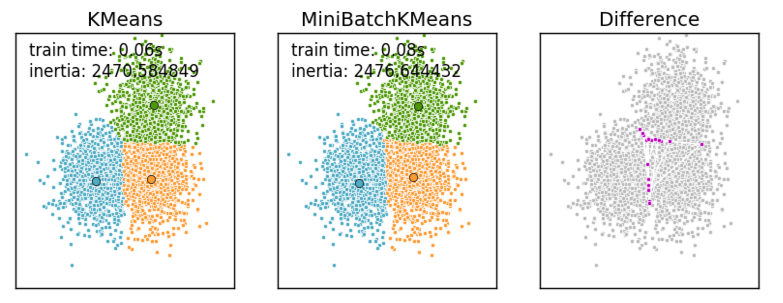6. K-Means与KNN

初学者很容易把K-Means和KNN搞混，两者其实差别还是很大的。

K-Means是无监督学习的聚类算法，没有样本输出；而KNN是监督学习的分类算法，有对应的类别输出。KNN基本不需要训练，对测试集里面的点，只需要找到在训练集中最近的k个点，用这最近的k个点的类别来决定测试点的类别。而K-Means则有明显的训练过程，找到k个类别的最佳质心，从而决定样本的簇类别。

当然，两者也有一些相似点，两个算法都包含一个过程，即找出和某一个点最近的点。两者都利用了最近邻(nearest neighbors)的思想。

列个表格对比一下特点：

KNN

K-Means

1.KNN是分类算法

2.监督学习

3.喂给它的数据集是带label的数据，已经是完全正确的数据

1.K-Means是聚类算法

2.非监督学习

3.喂给它的数据集是无label的数据，是杂乱无章的，经过聚类后才变得有点顺序，先无序，后有序

没有明显的前期训练过程，属于memory-based learning
有明显的前期训练过程
K的含义：来了一个样本x，要给它分类，即求出它的y，就从数据集中，在x附近找离它最近的K个数据点，这K个数据点，类别c占的个数最多，就把x的label设为c
K的含义：K是人工固定好的数字，假设数据集合可以分为K个簇，由于是依靠人工定好，需要一点先验知识

相似点：都包含这样的过程，给定一个点，在数据集中找离它最近的点。即二者都用到了NN(Nears Neighbor)算法，一般用KD树来实现NN。

7.应用实例

这是官网的一个例子，详细参见：http://scikit-learn.org/stable/auto_examples/cluster/plot_color_quantization.html#sphx-glr-auto-examples-cluster-plot-color-quantization-py

执行夏宫（中国）图像的逐像素矢量量化（VQ），将显示图像所需的颜色数量从96,615种独特颜色减少到64，同时保持整体外观质量。

在该示例中，像素在3D空间中表示，并且K均值用于找到64个颜色簇。在图像处理文献中，从K-means（聚类中心）获得的码本被称为调色板。使用单个字节，可以寻址多达256种颜色，而RGB编码每个像素需要3个字节。例如，GIF文件格式使用这样的调色板。

为了比较，还示出了使用随机码本（随机拾取的颜色）的量化图像。

print(__doc__)
import numpy as np
import matplotlib.pyplot as plt
from sklearn.cluster import KMeans
from sklearn.metrics import pairwise_distances_argmin
from sklearn.utils import shuffle
from time import time

n_colors = 64

# Load the Summer Palace photo

# Convert to floats instead of the default 8 bits integer coding. Dividing by
# 255 is important so that plt.imshow behaves works well on float data (need to
# be in the range [0-1])
china = np.array(china, dtype=np.float64) / 255

# Load Image and transform to a 2D numpy array.
w, h, d = original_shape = tuple(china.shape)
assert d == 3
image_array = np.reshape(china, (w * h, d))

print("Fitting model on a small sub-sample of the data")
t0 = time()
image_array_sample = shuffle(image_array, random_state=0)[:1000]
kmeans = KMeans(n_clusters=n_colors, random_state=0).fit(image_array_sample)
print("done in %0.3fs." % (time() - t0))

# Get labels for all points
print("Predicting color indices on the full image (k-means)")
t0 = time()
labels = kmeans.predict(image_array)
print("done in %0.3fs." % (time() - t0))

codebook_random = shuffle(image_array, random_state=0)[:n_colors + 1]
print("Predicting color indices on the full image (random)")
t0 = time()
labels_random = pairwise_distances_argmin(codebook_random,
image_array,
axis=0)
print("done in %0.3fs." % (time() - t0))

def recreate_image(codebook, labels, w, h):
"""Recreate the (compressed) image from the code book & labels"""
d = codebook.shape
image = np.zeros((w, h, d))
label_idx = 0
for i in range(w):
for j in range(h):
image[i][j] = codebook[labels[label_idx]]
label_idx += 1
return image

# Display all results, alongside original image
plt.figure(1)
plt.clf()
ax = plt.axes([0, 0, 1, 1])
plt.axis('off')
plt.title('Original image (96,615 colors)')
plt.imshow(china)

plt.figure(2)
plt.clf()
ax = plt.axes([0, 0, 1, 1])
plt.axis('off')
plt.title('Quantized image (64 colors, K-Means)')
plt.imshow(recreate_image(kmeans.cluster_centers_, labels, w, h))

plt.figure(3)
plt.clf()
ax = plt.axes([0, 0, 1, 1])
plt.axis('off')
plt.title('Quantized image (64 colors, Random)')
plt.imshow(recreate_image(codebook_random, labels_random, w, h))
plt.show()

第二个例子：

实例说明：利用sklearn.datasets.make_blobs产生1500条两维的数据集进行不同情况下的聚类示例，参见网址：https://blog.csdn.net/gamer_gyt/article/details/51244850

代码如下

import numpy as np      #科学计算包
import matplotlib.pyplot as plt      #python画图包

from sklearn.cluster import KMeans       #导入K-means算法包
from sklearn.datasets import make_blobs

plt.figure(figsize=(12, 12))

"""
make_blobs函数是为聚类产生数据集
产生一个数据集和相应的标签
n_samples:表示数据样本点个数,默认值100
n_features:表示数据的维度，默认值是2
centers:产生数据的中心点，默认值3
cluster_std：数据集的标准差，浮点数或者浮点数序列，默认值1.0
center_box：中心确定之后的数据边界，默认值(-10.0, 10.0)
shuffle ：洗乱，默认值是True
random_state:官网解释是随机生成器的种子
更多参数即使请参考：http://scikit-learn.org/dev/modules/generated/sklearn.datasets.make_blobs.html#sklearn.datasets.make_blobs
"""
n_samples = 1500
random_state = 170
X, y = make_blobs(n_samples=n_samples, random_state=random_state)

# Incorrect number of clusters
y_pred = KMeans(n_clusters=2, random_state=random_state).fit_predict(X)

plt.subplot(221)  #在2图里添加子图1
plt.scatter(X[:, 0], X[:, 1], c=y_pred) #scatter绘制散点
plt.title("Incorrect Number of Blobs")   #加标题

# Anisotropicly distributed data
transformation = [[ 0.60834549, -0.63667341], [-0.40887718, 0.85253229]]
X_aniso = np.dot(X, transformation)    #返回的是乘积的形式
y_pred = KMeans(n_clusters=3, random_state=random_state).fit_predict(X_aniso)

plt.subplot(222)#在2图里添加子图2
plt.scatter(X_aniso[:, 0], X_aniso[:, 1], c=y_pred)
plt.title("Anisotropicly Distributed Blobs")

# Different variance
X_varied, y_varied = make_blobs(n_samples=n_samples,
cluster_std=[1.0, 2.5, 0.5],
random_state=random_state)
y_pred = KMeans(n_clusters=3, random_state=random_state).fit_predict(X_varied)

plt.subplot(223)#在2图里添加子图3
plt.scatter(X_varied[:, 0], X_varied[:, 1], c=y_pred)
plt.title("Unequal Variance")

# Unevenly sized blobs
X_filtered = np.vstack((X[y == 0][:500], X[y == 1][:100], X[y == 2][:10]))
y_pred = KMeans(n_clusters=3, random_state=random_state).fit_predict(X_filtered)

plt.subplot(224)#在2图里添加子图4
plt.scatter(X_filtered[:, 0], X_filtered[:, 1], c=y_pred)
plt.title("Unevenly Sized Blobs")

plt.show() #显示图</span>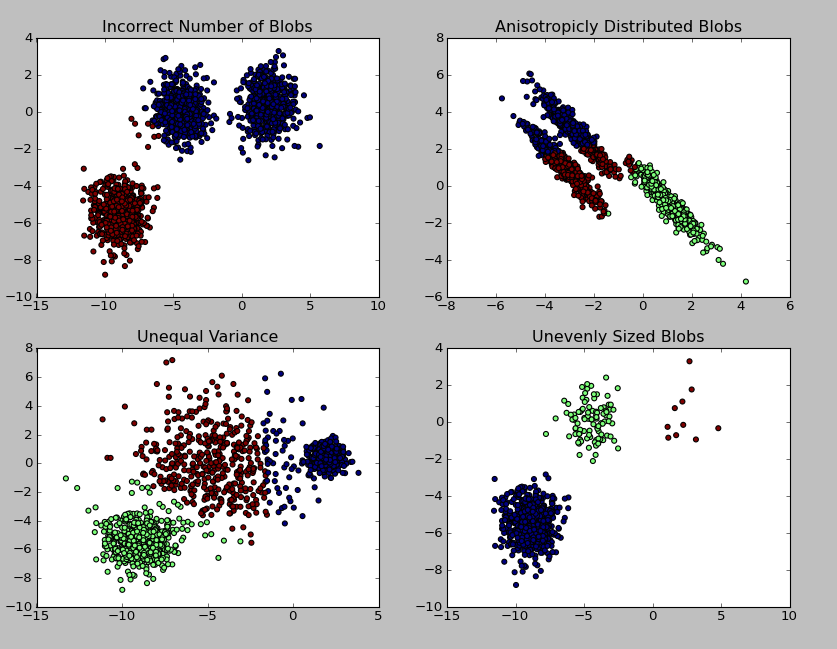参考网址：

https://www.cnblogs.com/pinard/p/6164214.html

https://blog.csdn.net/sinat_35512245/article/details/55051306

https://en.wikipedia.org/wiki/K-means_clustering

http://scikit-learn.org/stable/auto_examples/cluster/plot_color_quantization.html#sphx-glr-auto-examples-cluster-plot-color-quantization-py


展开全文K-means
• ## k-means 的原理，优缺点以及改进

万次阅读 多人点赞 2017-05-07 18:02:13
K-Means算法是无监督的聚类算法，它实现起来比较简单，聚类效果也不错，因此应用很广泛。K-Means算法有大量的变体，本文就从最传统的K-Means算法讲起，在其基础上讲述K-Means的优化变体方法。包括初始化优化K-Means+...机器学习
• 题目：本次作业的实验需求是使用分解聚类法与c-means聚类法对IRIS数据集进行聚类，Kmeans聚类代码网上摘录，分解聚类法纯原创，PS：因为时间紧，分解聚类法进行第二次分解时，偷懒了~~有缘人改改吧~~ 数据格式： ...python
• 在这里插入代码片@TOC 欢迎使用Markdown编辑器 你好！ 这是你第一次使用 Markdown编辑器 所展示的欢迎页。如果你想学习如何使用Markdown编辑器, 可以仔细阅读这篇文章，了解一下Markdown的基本语法知识。...python 聚类算法 机器学习 iris
•k-means
• ## K-Means（聚类）

万次阅读 多人点赞 2019-02-28 11:26:02
说到聚类，应先理解聚类和分类的区别，很多业务人员在日常分析时候不是很严谨，混为一谈，其实二者有本质的区别。 分类：分类其实是从特定的数据中挖掘模式，作出判断的过程。比如Gmail邮箱里有垃圾邮件分类器，...K-Means 聚类
• ## KMeans 算法（一）

万次阅读 多人点赞 2019-03-11 22:56:55
K-means算法简述 K-means算法，也称为K-平均或者K-均值，一般作为掌握聚类算法的第一个算法。 这里的K为常数，需事先设定，通俗地说该算法是将没有标注的 M 个样本通过迭代的方式聚集成K个簇。...
• ## 深入理解K-Means聚类算法

万次阅读 多人点赞 2016-12-18 20:50:09
概述什么是聚类分析聚类分析是在数据中发现数据对象之间的关系，将数据进行分组，组内的相似性越大，组间的差别越大，则聚类效果越好。不同的簇类型聚类旨在发现有用的对象簇，在现实中我们用到很多的簇的类型，使用...k-means 聚类算法
• ## 机器学习之k-means算法详解

万次阅读 多人点赞 2018-07-02 17:55:12
kmeans作用：去除奇异值 小结： 1-2 算法效果衡量标准 一、K值确定： 二、轮廓系数： 三、Canopy算法配合初始聚类： 1、Canopy简介： 2、Canopy+Kmeans： 四、Calinski-Harabasz Index： K-m...
• ## Kmeans聚类算法

万次阅读 2017-06-18 23:23:48
Kmeans聚类算法 动态聚类算法  任务：是将数据集划分成一定数量的子集，例如将一个数据集划分成3、4个子集等。因此要划分成多少个子集往往要预先确定，或大致确定，当然这个子集数目在理想情况能体现数据集比较...
• ## 从零开始实现Kmeans聚类算法

万次阅读 多人点赞 2017-11-01 14:30:09
声明：版权所有，转载请联系作者并注明出处: http://blog.csdn.net/u013719780?viewmode=contents知乎专栏： ...1. Kmeans聚类算法简介由于具有出色的速度和良好的可扩展性，机器学习 无监督学习
• ## KMeans聚类算法

千次阅读 2017-05-07 14:06:22
1、什么是聚类  所谓聚类就是将一组对象按照特征划分不为的小组，使得组内的差异性尽可能的小，组间的差异尽可能的大。例如，粗粒度的分类，按照学校实力，分为985、211高校，普通一本高校，二本高校，三本高校。...机器学习 python 实例
• Kmeans聚类算法C++ VS2010 调试运行
• kmeans聚类算法是一种简单实用的聚类算法，matlab自带函数kmeans可直接对数据进行kmeans聚类。为了方便更好地掌握kmeans聚类算法，今天我们自己来实现一个弱化的版本mykmeans。 mykmeans输入包含三项，分别为聚类所...matlab
• kmeans聚类算法介绍：kmeans算法是一种经典的无监督机器学习算法，名列数据挖掘十大算法之一。作为一个非常好用的聚类算法，kmeans的思想和实现都比较简单。kmeans的主要思想：把数据划分到各个区域(簇)，使得数据与...数据挖掘 matlab
• ## Kmeans聚类算法及其matlab源码

万次阅读 多人点赞 2016-10-24 14:57:34
本文介绍了K-means聚类算法，并注释了部分matlab实现的源码。MATLAB
• kmeans算法就是要将这个集合分成k类，每一类有一个中心，其中k的大小是人为设置的。 kmeans工作流程如下： 1. 随意选k个点作为每个类初始的中心点 2. 遍历每一个点i，分别计算点i到这k个点的距离，离哪一个点...
• 1.1 KMeans聚类算法 1.1.1 基础理论 KMeans算法的基本思想是初始随机给定K个簇中心，按照最邻近原则把待分类样本点分到各个簇。然后按平均法重新计算各个簇的质心，从而确定新的簇心。一直迭代，直到簇心的移动距离...spark mllib
• 自己实现kmeans聚类算法，matlab语言，带有界面，容易理解matlab 界面 数据
• Kmeans聚类算法详解与实现，Kmeans算法的MATLAB实现、python实现源代码都有。附有算法原理的解析。 对应的博客地址：http://blog.csdn.net/zengxiantao1994/article/details/73441922
• ## kmeans聚类算法

千次阅读 2018-08-21 18:24:14
本文记录kmeans聚类算法学习的相关内容，后续会更新............ 概念：kmeans算法是输入聚类个数k，以及包含 n个数据对象的数据库，输出满足方差最小标准k个聚类的一种算法。k-means 算法将n个数据对象划分为 k个...
• 机器学习实战——Kmeans聚类算法1 聚类算法介绍2 sklearn中的实现 1 聚类算法介绍 在无监督学习中，训练样本的标记是未知的，目标是通过对无标记训练样本的学习来揭示数据的内在性质及规律，为进一步的数据分析...机器学习 K均值聚类
• 本篇博客，我们将结合当前阶段正在做的用户画像项目，为大家介绍RFM模型和KMeans聚类算法。         先赞后看，养成习惯！ 文章目录一、RFM模型引入1、RFM详解1.1 R值：...用户画像 RFM模型 机器学习
• 文章目录Kmeans聚类算法及应用1. Kmeans聚类算法原理1.2 算法图示1.3 算法要点1.3.1 核心思想1.3.2 算法步骤图解1.3.3 算法实现步骤2. Kmeans分类算法Python实战2.1 需求2.2 python代码实现2.2.1 利用numpy手动实现...python
• 机器学习算法-KMeans聚类算法解析及伪代码实现。 徐小狗在文末附上了几条大神们关于KMeans聚类算法的博文，欲详细研究请前往浏览~ 作为初学者，许多地方可能笨拙或有误，希望有大神看到后给予优化和指点！~ ...机器学习 伪代码
• Kmeans聚类算法及其 Python实现Kmeans聚类算法及其 Python实现 关于聚类 基本思想 初始质心的选择 算法实验 Python实现 本节内容：本节内容是根据上学期所上的模式识别课程的作业整理而来，第一道题目是Kmeans聚类...python...